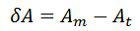# Static Error

Definition: A static error is defined as the difference between the measured value and the true value of the quantity. The true value is the exact value of the measurement which is impossible to obtain. Hence the approximate true value of the measurement should be taken into consideration.

The accuracy is the most important characteristic of an instrument, and it is measured regarding errors. The instrument is said to be accurate if their measured value is nearby its true value.

The errors mainly occur because of poor design and improper maintenance of the system. Human error, systematic error and random error are the types of static error. The human error occurs because of human mistakes, for example, error in reading or because of instrument measurement, etc. The systematic error occurs because of the instrument defect, and the random error is caused because of unpredictable reason like the variation from one reading to another, etc.

## Formula of Static Error

The static error is represented by the formula shown below.Where δA = error
Am = measured value of quantity
At = true value of quantity

δA is also called the absolute static error of quantity A.Where ∈0 = absolute static error of quantity A under measurement.

The absolute value of A does not indicate precisely the accuracy of measurements. For example, Consider the error of A is negligible when the current being measured is of the order of 1000 A while the same error of A is intolerable when the current under measurement is 10 Amp or so.

Thus the quality of measurement is provided by the relative static error, i.e., the ratio of absolute static error A to At, the true value of the quantity under measurement.Therefore the relative static error is given byPercentage static error is given by the equation shown belowWhen the absolute static error is small which means that the difference between the true and measured value is small, ∈<<1.